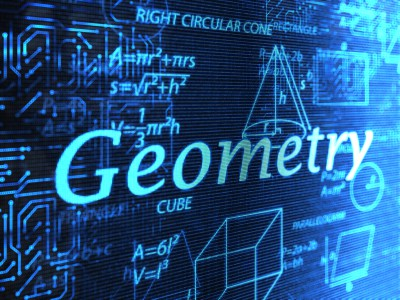# Quant Quiz on “GEOMETRY” for SSC CGL 2016

For the first time SSC 2016 examination will be held in Computer Based pattern and managing time will be one of the important factor while solving the questions. So in order to make students familiar with such a situations we are providing questions in a time based manner , which will help students to manage the time properly.1. The altitude drawn to the base of an iscoceles triangle is 8 cm and its permineter is 64 cm then find the area of triangle.

120 cm^2
180 cm^2
125 cm^2
170 cm^2

2. The perimeter of an isocles triangle is 544 cm and each of equal side is 5/6th of the base then find the area of triangle?

12672 cm^2
13872 cm^2
13121 cm^2
11892 cm^2

3. If two chords of a circle with length 2a and 2b intersect each other at right angle. Then find the radius of circle if the distance between centre of circle and point of intersection of chord is ‘c’.

a
b
c
d

4. QR and QP are two chords of a circle with centre at O. and QR = QP. Two tangents are drawn at P and Q which intersect at C. If ∠RQP=68° find angle ∠QOP and ∠QCP.

112°,68°
105°,75°
100°,80°
92°,88°

5. ABC and MNC are two secant of a circle which intersect each other outside the circle. Line BM is the diameter of the circle the find the value of ∠AMB and ∠ANBif ∠c=32° and ∠BMN=40°

18°,32°
18°,18°
20°,25°
None of the above

6. Assume that a drop of water is spherical and tis diameter is 1/10 of a centimeter. A conical glass has a height equal to diameter of its rim. If 32000 drops of water fill the glass completely then the height of glass in cm is –

8
4
6
5

7. A conical cup filled with ice cream. The ice-cream form a hemispherical shape on its open top. The height of hemispherical part is 7 cm and radius of the hemispherical part equal to the height of the cone then the volume of ice-cream is –

1078 cm^3
1708 cm^3
7108 cm^3
7180 cm^3

8. The sum of length, breadth and height of a cuboid is 38 cm and length of its diagonal is 22 cm. Find the surface are (T. S. A.).

960 cm^2
1024 cm^2
884 cm^2
676 cm^2

9.  A ball of lead 4 cm in a diameter is covered with gold if the volume of gold and lead are equal then the thickness of gold is -[∛2=1.259]

.602 cm
0.518 cm
.419 cm
0.712 cm

10. Semi vertical angle of a cone is 30° and slant height is 4 cm. Then find the volume of cone.

a
b
c
d

×

## Download success!

Thanks for downloading the guide. For similar guides, free study material, quizzes, videos and job alerts you can download the Adda247 app from play store.

Thank You, Your details have been submitted we will get back to you.

## Leave a comment

×
Login
OR

Forgot Password?

×
Sign Up
ORForgot Password
Enter the email address associated with your account, and we'll email you an OTP to verify it's you.Reset Password
Please enter the OTP sent to
/6

×
CHANGE PASSWORD